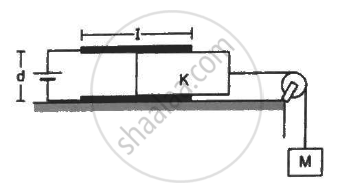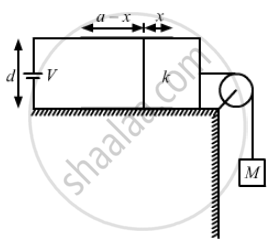Department of Pre-University Education, Karnataka course PUC Karnataka Science Class 12
Share

# Consider the Situation Shown in Figure (31-e29). the Width of Each Plate is B. the Capacitor - Physics

ConceptPotential Due to an Electric Dipole

#### Question

Consider the situation shown in figure (31-E29). The width of each plate is b. The capacitor plates are rigidly clamped in the laboratory and connected to a battery of emf ε. All surfaces are frictionless. Calculate the value of M for which the dielectric slab will stay in equilibrium.#### SolutionLet the potential of the battery connected to the capacitor be V.

Let the length of the part of the slab inside the capacitor be x.

The capacitor can be considered to be two capacitors in parallel.

The capacitances of the two capacitors in parallel are :-

C_1 = (K_1∈_0bx)/d , C_2 = (∈_0b(l_1 - x))/d

C1 is the part of the capacitor having the dielectric inserted in it and C2 is the capacitance of the part of the capacitor without dielectric.

As, C1 and C2 are in parallel.

Therefore, the net capacitance is given by

C = C_1 + C_2

C = (K_1∈_0bx)/d + (∈_0b(l_1 - x))/d

C = (∈_0b)/d [l_1 + x(K_1 - 1)]

The dielectric slab is attracted by the electric field of the capacitor and applies a force in left direction.

Let us consider electric force of magnitude F pulls the slab in left direction.

Let there be an infinitesimal displacement dx in left direction by the force F.

The work done by the force = F.dx

Let the potential of the battery connected to the left capacitor be V1 and that of the battery connected with the right capacitor be V2.

With the displacement of slab, the capacitance will increase, therefore the energy of the capacitor will also increase. In order to maintain constant voltage, the battery will supply extra charges, therefore the battery will do work.

Work done by the battery = change in energy of capacitor + work done by the force F on the capacitor

dW_B = dU + dW_F

Let the charge dq is supplied by the battery, and the change in the capacitor be dC

dW_B = (dq).V = (dC).V^2

dU = 1/2(dC). V^2

(dC). V^2 = 1/2 (dC). V^2 + F.dx

1/2 (dC). V^2 = F.dx

⇒ F = 1/2 (dC)/dx V_1^2

⇒ F = 1/2 d/dx ((∈_0b)/d[l_1 + x(K_1 - 1)])V_1^2

⇒ F= (∈_0bV_1^2(K_1 - 1))/(2d)

To keep the dielectric slab in equilibrium, the electrostatic force on it must be counteracted by the weight of the block attached.

Therefore,

(∈_0bV^2)/(2d)(K-1) = Mg

⇒ M = (∈_0bV^2)/(2dg)(K-1)

Is there an error in this question or solution?

#### APPEARS IN

Solution Consider the Situation Shown in Figure (31-e29). the Width of Each Plate is B. the Capacitor Concept: Potential Due to an Electric Dipole.
S